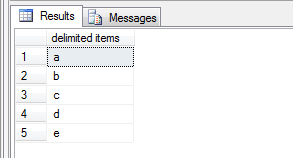## Apr 22, 2011## Columns to Rows in SQL Server

In this article, you will learn how to convert columns to rows in SQL Server.

We will use unpivot feature for the transformation. Lets' take an example

NOTE: PIVOT/UNPIVOT feature was introduced in SQL Server 2005, so solution will work in SQL Server 2005 onward only.

Let's create a table for Key Value pair and populate some data in it
```CREATE TABLE tblkeyvalpair(
KEY1 INT, KEY2 INT, KEY3 INT, KEY4 INT, KEY5 INT
)
INSERT INTO tblkeyvalpair VALUES (10, 20, 30, 40, 50)

SELECT * FROM tblkeyvalpair```
OUTPUT

Here, the keys and values are stored in column form, and our requirement is to display the key and value pair in row form.

Below is the code snippet to achieve the same using unpivot feature
```SELECT KeyName, Value
FROM   (
SELECT * FROM   tblkeyvalpair
) AS t1
UNPIVOT (
Value FOR KeyName IN (key1, key2, key3, key4, key5)
) AS t2```
If we know all key columns then we can hard code the values as we do in the above solution

But now assume we don't all possible values, in that case we will have to dynamically construct the list of keys and pass it dynamically to the query.
```DECLARE @ColumnList VARCHAR(MAX),
@Query      VARCHAR(MAX),
@ColumnName VARCHAR(100),
@TableName  NVARCHAR(100)

SET @TableName = 'tblkeyvalpair'

SELECT @ColumnList = Stuff((SELECT ',' + name
FROM   syscolumns
WHERE  id = Object_id(@TableName)
FOR XML PATH(''))
,1,1,'')

SET @Query = 'SELECT keyname, value FROM(SELECT * FROM ' + @TableName + ') AS T1'
SET @Query = @Query + ' UNPIVOT (value FOR keyname IN (' + @ColumnList + ')) AS T2'

EXEC(@Query)```
OUTPUT

## Apr 17, 2011## Display Comma Separated Value Row Wise in SQL Server

In this post, we will learn how to display comma separated value row wise in SQL Server

Example-1: Comma separated values of a variable to rows
In the first Example we will take a variable which holds some values with comma separator and we will display each value in a row.

Step 1: Replace comma with tag from comma separated value list to make it as XML
Step 2: Read each node from XML and display value in rows

```DECLARE @xml XML, @s NVARCHAR(MAX)
SELECT  @s = N'a,b,c,d,e'
SET     @xml = N'<t>' + REPLACE(@s,',','</t><t>') + '</t>'
SELECT  t.value('.','varchar(5)') as [delimited items]
FROM    @xml.nodes('/t') as a(t)```
OUTPUT

Example-2: Comma separated values of a column to rows
In this example, we have two columns Col1 & Col2 in a table and Col2 stores comma separated values and our requirement is to display each value of Col2 in row form against Col1

Step 1: Replace comma with tag from comma separated value list to make it as XML
Step 2: Reading each node from the XML
Step 3: Cross Apply to generated each node value as row.

Suggested article to understand Cross Apply: APPLY Operator in SQL Server

```DECLARE @TAB TABLE (col1 varchar(10), col2 varchar(10))
INSERT INTO @TAB
SELECT 'A' as col1, '1,2,3' as col2
UNION
SELECT 'B' as col1, '4,5,6' as col2

SELECT col1, Tags.val.value('.', 'VARCHAR(MAX)') AS col2
FROM(
SELECT  col1, CAST('<t>' + REPLACE(col2, ',', '</t><t>') + '</t>' AS XML) AS TAG
FROM    @TAB
) T CROSS APPLY TAG.nodes('/t') as Tags(val)```
OUTPUT

Now let's transform the above code into a table valued function to make it reusable and flexible.

```CREATE FUNCTION Split(
@delimited NVARCHAR(MAX),
@delimiter NVARCHAR(100)
) RETURNS @t TABLE (id INT IDENTITY(1,1), val NVARCHAR(MAX))
AS
BEGIN
DECLARE @xml XML
SET @xml = N'<t>' + REPLACE(@delimited,@delimiter,'</t><t>') + '</t>'
INSERT INTO @t(val)
SELECT  r.value('.','varchar(MAX)') as item
FROM  @xml.nodes('/t') as records(r)
RETURN
END```

Now, In the above table valued function we have to just pass delimited string and delimiter and it will return you a table with two columns id (sequential number) and val (all delimited value).
Delimiter could be any : Comma, Colon, Semi-Colon etc.

Suggested article to understand split function in detail: Split Function in SQL

Now, let's execute the table valued function with different delimiters

```SELECT * FROM dbo.Split(N'a,b,c', ',')
SELECT * FROM dbo.Split(N'p:q:r', ':')```
OUTPUT